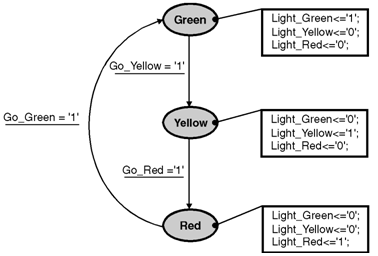Home > finite state machines > FSM Applications > Simple Traffic Controller

# Simple-Traffic-Controller

Simple Traffic Controller : Let us conider the operation of traffic lights controller. In this case when the red light is on and timer signals that makes green light should be glow (GO_GREEN signal goes to high state) and hence turns on the GREEN signal and makes off the other signals. When green light is on and timer signals makes the yellow light GO_YELLOW signal is in high state) makes on the YELLOW signal and makes other signals off. When the yellow light is on and timer signals that the red light makes the (GO_RED signal in high state) makes on the RED signal and other signals off. As an example, a State diagram for traffic lights controller is shown in figure below.A VHDL description of a traffic lights controller is shown below :

```    entity traf is
port (CLK: in STD_LOGIC; GO_GREEN: in STD_LOGIC;
GO_RED: in STD_LOGIC; GO_YELLOW: in STD_LOGIC;
LIGHT_GREEN: out STD_LOGIC; LIGHT_RED: out STD_LOGIC;
.	LIGHT_YELLOW: out STD_LOGIC
);
end traf
architecture arch of traf is
type LIGHTS_type is (GREEN, RED, YELLOW);
signal LIGHTS: LIGHTS_type;
begin
process (CLK)
begin
if CLK'event and CLK = '1' then
case LIGHTS is
when GREEN =>    if GO_YELLOW='1' then LIGHTS <= YELLOW;
end if;
when RED =>    	if GO_GREEN='1' then LIGHTS <= GREEN;
end if;
when YELLOW => if GO_RED='1' then LIGHTS <= RED;
.					end if;
when others => null;
end case;
end if;
end process;
LIGHT_GREEN <= '1' when (LIGHTS = GREEN) else
'0' when (LIGHTS = RED) else
'0' when (LIGHTS = YELLOW) else '0';
LIGHT_YELLOW <= '0' when (LIGHTS = GREEN) else
'0' when (LIGHTS = RED) else
'1' when (LIGHTS = YELLOW) else   '1';
LIGHT_RED <= '0' when (LIGHTS = GREEN) else
'1' when (LIGHTS = RED) else
'0' when (LIGHTS = YELLOW) else  '0';
end arch;

```